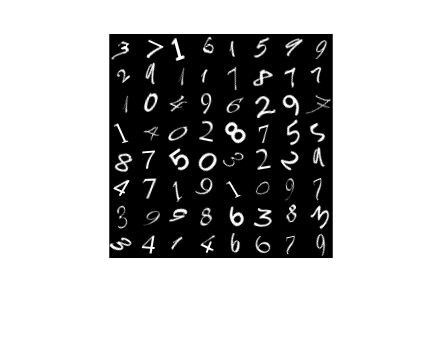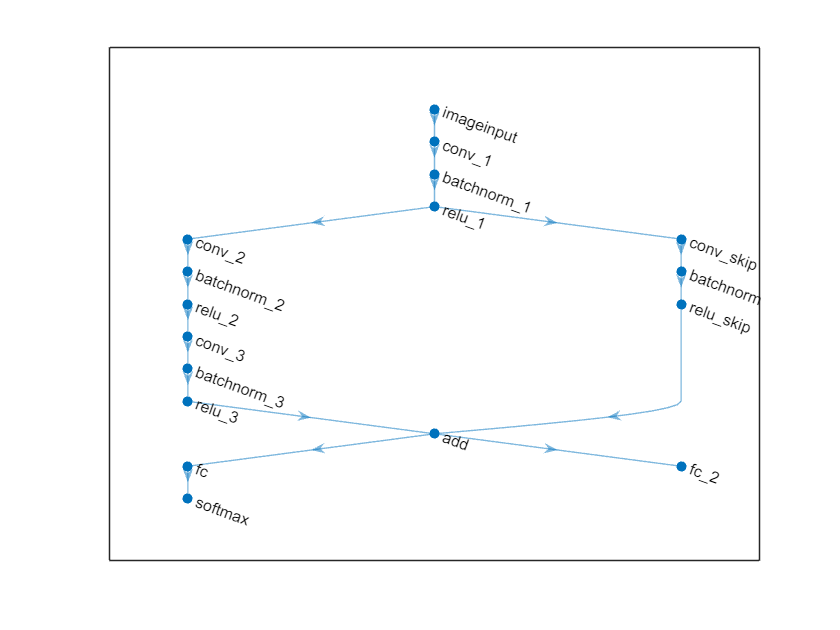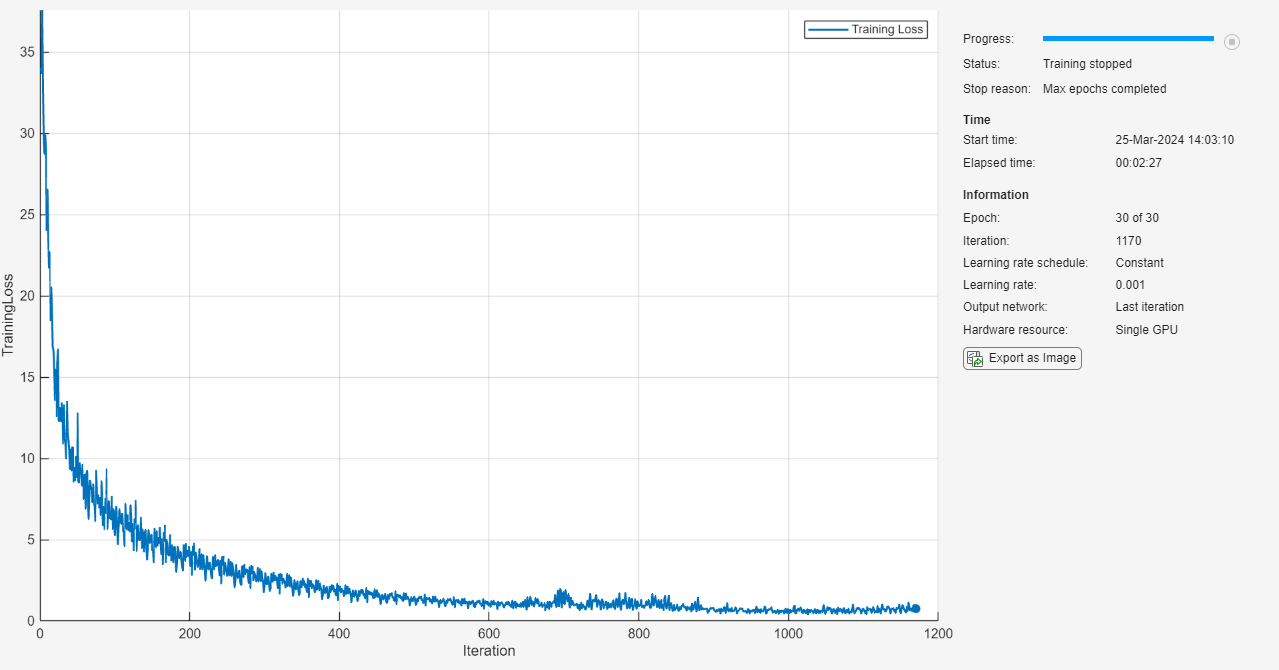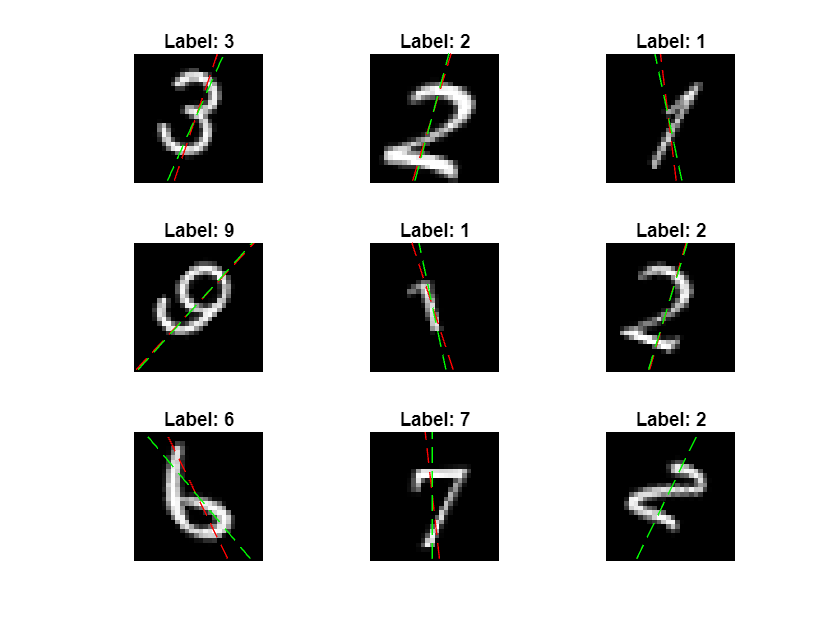# Train Network with Multiple Outputs

This example shows how to train a deep learning network with multiple outputs that predict both labels and angles of rotations of handwritten digits.

To train a network with multiple outputs, you must train the network using a custom training loop.

The `digitTrain4DArrayData` function loads the images, their digit labels, and their angles of rotation from the vertical. Create an `arrayDatastore` object for the images, labels, and the angles, and then use the `combine` function to make a single datastore that contains all of the training data. Extract the class names and number of nondiscrete responses.

```[XTrain,T1Train,T2Train] = digitTrain4DArrayData; dsXTrain = arrayDatastore(XTrain,IterationDimension=4); dsT1Train = arrayDatastore(T1Train); dsT2Train = arrayDatastore(T2Train); dsTrain = combine(dsXTrain,dsT1Train,dsT2Train); classNames = categories(T1Train); numClasses = numel(classNames); numObservations = numel(T1Train);```

View some images from the training data.

```idx = randperm(numObservations,64); I = imtile(XTrain(:,:,:,idx)); figure imshow(I)```### Define Deep Learning Model

Define the following network that predicts both labels and angles of rotation.

• A convolution-batchnorm-ReLU block with 16 5-by-5 filters.

• Two convolution-batchnorm-ReLU blocks each with 32 3-by-3 filters.

• A skip connection around the previous two blocks containing a convolution-batchnorm-ReLU block with 32 1-by-1 convolutions.

• Merge the skip connection using addition.

• For classification output, a branch with a fully connected operation of size 10 (the number of classes) and a softmax operation.

• For the regression output, a branch with a fully connected operation of size 1 (the number of responses).

Define the main block of layers as a layer graph.

```layers = [ imageInputLayer([28 28 1],Normalization="none") convolution2dLayer(5,16,Padding="same") batchNormalizationLayer reluLayer(Name="relu_1") convolution2dLayer(3,32,Padding="same",Stride=2) batchNormalizationLayer reluLayer convolution2dLayer(3,32,Padding="same") batchNormalizationLayer reluLayer additionLayer(2,Name="add") fullyConnectedLayer(numClasses) softmaxLayer(Name="softmax")]; lgraph = layerGraph(layers);```

```layers = [ convolution2dLayer(1,32,Stride=2,Name="conv_skip") batchNormalizationLayer reluLayer(Name="relu_skip")]; lgraph = addLayers(lgraph,layers); lgraph = connectLayers(lgraph,"relu_1","conv_skip"); lgraph = connectLayers(lgraph,"relu_skip","add/in2");```

Add the fully connected layer for regression.

```layers = fullyConnectedLayer(1,Name="fc_2"); lgraph = addLayers(lgraph,layers); lgraph = connectLayers(lgraph,"add","fc_2");```

View the layer graph in a plot.

```figure plot(lgraph)```Create a `dlnetwork` object from the layer graph.

`net = dlnetwork(lgraph)`
```net = dlnetwork with properties: Layers: [17×1 nnet.cnn.layer.Layer] Connections: [17×2 table] Learnables: [20×3 table] State: [8×3 table] InputNames: {'imageinput'} OutputNames: {'softmax' 'fc_2'} Initialized: 1 View summary with summary. ```

#### Define Model Loss Function

Create the function `modelLoss`, listed at the end of the example, that takes as input, the `dlnetwork` object, a mini-batch of input data with corresponding targets containing the labels and angles, and returns the loss, the gradients of the loss with respect to the learnable parameters, and the updated network state.

### Specify Training Options

Specify the training options. Train for 30 epochs using a mini-batch size of 128.

```numEpochs = 30; miniBatchSize = 128;```

### Train Model

Use `minibatchqueue` to process and manage the mini-batches of images. For each mini-batch:

• Use the custom mini-batch preprocessing function `preprocessMiniBatch` (defined at the end of this example) to one-hot encode the class labels.

• Format the image data with the dimension labels `"SSCB"` (spatial, spatial, channel, batch). By default, the `minibatchqueue` object converts the data to `dlarray` objects with underlying type `single`. Do not add a format to the class labels or angles.

• Train on a GPU if one is available. By default, the `minibatchqueue` object converts each output to a `gpuArray` if a GPU is available. Using a GPU requires Parallel Computing Toolbox™ and a supported GPU device. For information on supported devices, see GPU Computing Requirements (Parallel Computing Toolbox).

```mbq = minibatchqueue(dsTrain,... MiniBatchSize=miniBatchSize,... MiniBatchFcn=@preprocessData,... MiniBatchFormat=["SSCB" "" ""]);```

Train the model using a custom training loop. For each epoch, shuffle the data and loop over mini-batches of data. At the end of each iteration, display the training progress. For each mini-batch:

• Evaluate the model loss and gradients using `dlfeval` and the `modelLoss` function.

• Update the network parameters using the `adamupdate` function.

```trailingAvg = []; trailingAvgSq = [];```

Calculate the total number of iterations for the training progress monitor

```numIterationsPerEpoch = ceil(numObservations / miniBatchSize); numIterations = numEpochs * numIterationsPerEpoch;```

Initialize the `TrainingProgressMonitor` object. Because the timer starts when you create the monitor object, make sure that you create the object close to the training loop.

```monitor = trainingProgressMonitor( ... Metrics="Loss", ... Info="Epoch", ... XLabel="Iteration");```

Train the model.

```epoch = 0; iteration = 0; while epoch < numEpochs && ~monitor.Stop epoch = epoch + 1; % Shuffle data. shuffle(mbq) % Loop over mini-batches. while hasdata(mbq) && ~monitor.Stop iteration = iteration + 1; [X,T1,T2] = next(mbq); % Evaluate the model loss, gradients, and state using % the dlfeval and modelLoss functions. [loss,gradients,state] = dlfeval(@modelLoss,net,X,T1,T2); net.State = state; % Update the network parameters using the Adam optimizer. [net,trailingAvg,trailingAvgSq] = adamupdate(net,gradients, ... trailingAvg,trailingAvgSq,iteration); % Update the training progress monitor. recordMetrics(monitor,iteration,Loss=loss); updateInfo(monitor,Epoch=epoch + " of " + numEpochs); monitor.Progress = 100*iteration/numIterations; end end```### Test Model

Test the classification accuracy of the model by comparing the predictions on a test set with the true labels and angles. Manage the test data set using a `minibatchqueue` object with the same setting as the training data.

```[XTest,T1Test,T2Test] = digitTest4DArrayData; dsXTest = arrayDatastore(XTest,IterationDimension=4); dsT1Test = arrayDatastore(T1Test); dsT2Test = arrayDatastore(T2Test); dsTest = combine(dsXTest,dsT1Test,dsT2Test); mbqTest = minibatchqueue(dsTest,... MiniBatchSize=miniBatchSize,... MiniBatchFcn=@preprocessData,... MiniBatchFormat=["SSCB" "" ""]);```

To predict the labels and angles of the validation data, loop over the mini-batches and use the `predict` function. Store the predicted classes and angles. Compare the predicted and true classes and angles and store the results.

```classesPredictions = []; anglesPredictions = []; classCorr = []; angleDiff = []; % Loop over mini-batches. while hasdata(mbqTest) % Read mini-batch of data. [X,T1,T2] = next(mbqTest); % Make predictions using the predict function. [Y1,Y2] = predict(net,X,Outputs=["softmax" "fc_2"]); % Determine predicted classes. Y1 = onehotdecode(Y1,classNames,1); classesPredictions = [classesPredictions Y1]; % Dermine predicted angles Y2 = extractdata(Y2); anglesPredictions = [anglesPredictions Y2]; % Compare predicted and true classes T1 = onehotdecode(T1,classNames,1); classCorr = [classCorr Y1 == T1]; % Compare predicted and true angles angleDiffBatch = Y2 - T2; angleDiffBatch = extractdata(gather(angleDiffBatch)); angleDiff = [angleDiff angleDiffBatch]; end```

Evaluate the classification accuracy.

`accuracy = mean(classCorr)`
```accuracy = 0.9882 ```

Evaluate the regression accuracy.

`angleRMSE = sqrt(mean(angleDiff.^2))`
```angleRMSE = single 6.3569 ```

View some of the images with their predictions. Display the predicted angles in red and the correct labels in green.

```idx = randperm(size(XTest,4),9); figure for i = 1:9 subplot(3,3,i) I = XTest(:,:,:,idx(i)); imshow(I) hold on sz = size(I,1); offset = sz/2; thetaPred = anglesPredictions(idx(i)); plot(offset*[1-tand(thetaPred) 1+tand(thetaPred)],[sz 0],"r--") thetaValidation = T2Test(idx(i)); plot(offset*[1-tand(thetaValidation) 1+tand(thetaValidation)],[sz 0],"g--") hold off label = string(classesPredictions(idx(i))); title("Label: " + label) end```### Model Loss Function

The `modelLoss` function, takes as input, the `dlnetwork` object `net`, a mini-batch of input data `X` with corresponding targets `T1` and `T2` containing the labels and angles, respectively, and returns the loss, the gradients of the loss with respect to the learnable parameters, and the updated network state.

```function [loss,gradients,state] = modelLoss(net,X,T1,T2) [Y1,Y2,state] = forward(net,X,Outputs=["softmax" "fc_2"]); lossLabels = crossentropy(Y1,T1); lossAngles = mse(Y2,T2); loss = lossLabels + 0.1*lossAngles; gradients = dlgradient(loss,net.Learnables); end```

### Mini-Batch Preprocessing Function

The `preprocessMiniBatch` function preprocesses the data using the following steps:

1. Extract the image data from the incoming cell array and concatenate into a numeric array. Concatenating the image data over the fourth dimension adds a third dimension to each image, to be used as a singleton channel dimension.

2. Extract the label and angle data from the incoming cell arrays and concatenate along the second dimension into a categorical array and a numeric array, respectively.

3. One-hot encode the categorical labels into numeric arrays. Encoding into the first dimension produces an encoded array that matches the shape of the network output.

```function [X,T1,T2] = preprocessData(dataX,dataT1,dataT2) % Extract image data from cell and concatenate X = cat(4,dataX{:}); % Extract label data from cell and concatenate T1 = cat(2,dataT1{:}); % Extract angle data from cell and concatenate T2 = cat(2,dataT2{:}); % One-hot encode labels T1 = onehotencode(T1,1); end```